Parası kalmadığı için otobüse binemiyordur ailesi porno izle ona daha yeni para gönderdiği için tekrar porno istemeye utanınca mecburen otostop çekmek için youporn çantasını alarak yol kenarına gelir etekli porno liseli türk kız yol kenarında dururken yanına yaklaşan porno kibar bir gencin onu gideceği yere kadar bırakmak porno izle istemesine çok mutlu olur arabaya bindiklerinde gideceği yer ile porno arabayı kullanan adamın gittiği yer arasında çok mesafe sex izle farkı olduğunu anlayan türk kız bu yaptığı porno indir iyilik karşısında arabada ona memelerini açar porno sapıklaşan adam yol kenarındaki hotelde durarak porno izle üniversiteli otostop çeken türk kızına odada sakso çektirip sikerTheoretical Study of Spherical Langmuir Probe in Maxwellian Plasma
All submissions of the EM system will be redirected to Online Manuscript Submission System. Authors are requested to submit articles directly to Online Manuscript Submission System of respective journal.

Original Article
, Volume: 5( 1)

# Theoretical Study of Spherical Langmuir Probe in Maxwellian Plasma

*Corresponding Author:
Bhattarai S
MSc Physics, Department of Physics, Patan Multiple Campus, Tribhuvan University, Lalitpur, Nepal Tel: 74951376111, E-mail: [email protected]

Received Date: March 27, 2017 Accepted Date: March 27, 2017 Published Date: April 4, 2017

Citation: Bhattarai S, Mishra LN. Theoretical Study of Spherical Langmuir Probe in Maxwellian Plasma. J Phys Astron. 2017;5(1):107.

### Abstract

The Langmuir Probe is the key plasma diagnostic used by scientists interested in plasma characterization to measure the internal parameters of the bulk of the plasma. Spherical Langmuir Probes have been installed on satellites and sounding rockets to observe the general characteristics of thermal plasma in the ionosphere for more than five decades. Because of its simplicity and convenience, the Langmuir probe is one of the most frequently installed scientific instruments on spacecraft. This research explores the theoretical study of Spherical Langmuir Probe I-V Characteristics. With the help of the (volt–ampere curves) of spherical Langmuir probes, the different parameters of plasma can be determined such as plasma potential, floating potential, probe currents in different probe voltage and so on. The effect of electron temperature on the Electron Energy Distribution Function (EEDF) was also analyzed. At higher energy range, the shape of the distribution recovers and the tail trend with energy is maintained and decreases exponentially.

### Keywords

Langmuir probe; Plasma parameters; I-V characteristics; Maxwellian plasma

### Introduction

Langmuir probe as a plasma diagnostic device has been widely used to characterize laboratory plasmas and is accepted worldwide for the measurement of plasma parameters like electron density, electron temperature, floating potential, plasma potential, and electron energy distribution. An electrostatic probe was first used to measure the potential distribution in gas discharges on the ground by J.J. Thomson. The theory was later developed by Langmuir and his collaborators . The technique, with further developments, has been extensively applied to the study of gas discharges and also to the study of the ionosphere. A Langmuir probe refers to an electrode immersed in charged particle plasma, whose current - voltage (I-V) characteristics can be measured . From the I-V characteristics, one can estimate the temperature and number density of thermal electrons as bulk parameters. The technique has been used to measure thermal plasma populations on spacecraft in the ionosphere, although the conditions are more complex on a fast moving platform. The first in situ measurement of electron temperature in the ionosphere was made by Langmuir probe in 1947 .

There is no general theory of Langmuir probes which is applicable to all measurement conditions, because it depends on the probe size and geometry, plasma density and temperature, platform velocity, and other factors. The actual design of the probe is usually determined by considering the relationship between the probe dimensions and the Debye length of the plasma. In general, two approximations are used to express the current on the probe in the plasma: 1) Orbital Motion Limited (OML) and 2) Sheath Area Limited (SAL). OML theory can be adopted when the probe radius is smaller than the thickness of the sheath surrounding the probe, while it must be equal to or larger than the sheath thickness in the case of SAL theory.

### Langmuir Probe Description

Although the working principle of the Langmuir probe is bit complex, its construction is quite simple. The probe tip is made up of high temperature material like tungsten, molybdenum, platinum, or nickel, which can withstand high temperature. Frequently used geometries of Langmuir probes are planar, spherical, and cylindrical shapes.

Probe type and dimensions

The geometry is chosen depending on the purpose of the measurements and the platform configuration. We most commonly adopt the cylindrical geometry because this allows the probe radius to be small enough to satisfy the OML condition for usual ionospheric conditions, while the length can be long so that the surface area can be increased. This enables us to collect sufficient current under the OML condition even at low electron densities. For a spherical probe, it is not easy to get the same amount of current without breaking the OML condition for the same ionospheric conditions, because the diameter of the probe has to be increased.

Langmuir probes may have different electrode shapes, such as cylindrical, spherical, and planar probes. The cylindrical probe is a straight piece of wire typically made from tantalum or similar materials such as molybdenum, which are chosen for their high melting points and mechanical strength; tungsten and graphite are also popular choices. In contrast, the planar probe is a flat conductor that is typically single-sided, with the rest of the probe being insulated with a simple glass sleeve or coaxial cables built into the sleeve (Figure. 1).

Figure 1: An illustration of different types of collecting Langmuir probes with radius rp; a) Spherical, b) Cylindrical and c) Planar geometries .

### Langmuir Probe Installation

Several factors have to be considered in determining where a Langmuir probe should be installed on a satellite. The use of a boom is required for the probe to conduct measurements beyond the spacecraft sheath and outside the disturbed region (wake) caused by the satellite movements. When the spacecraft is significantly charged either negatively or positively, a sheath will develop around its surface, which can affect the probe’s measurements. If the Langmuir probe is inside the sheath of a negatively charged spacecraft, the probe characteristics may be modified compared to outside the sheath . They suggested that the probe characteristics are likely to depart from the usual OML theory, having a detrimental effect on the process of extracting plasma parameters from measured current-voltage (I-V) curves. Since the Debye length increases as the electron temperature increases or the electron density decreases, care must be taken regarding the sheath effect when using a Langmuir probe on such situation.

Derivation of electron density and temperature

When a probe is immersed in plasmas, the probe current generally depends on the collections of positive ions, negative ions, and electrons. We consider the electron current on a spherical probe under the condition that the electrons have a Maxwellian velocity distribution in a coordinate system fixed with respect to the probe.

Figure. 2 shows the current-voltage (I-V) characteristics obtained by sweeping the probe voltage, Vp, with respect to the spacecraft potential, Vs, while measuring the net current, I, which consists of the ion current, Ii, and the electron current, Ie. The I-V characteristics has three different regions; 1) ion saturation region where the electrons are repelled but ions are collected, 2) electron retarding potential region where most of the current is due to electrons, but the actual current is determined by the number of electrons which can overcome a retarding potential Vr (=VsVp), and 3) electron saturation region where ions are repelled but electrons are attracted to the probe. The increase in the probe current in the electron saturation region is due to sheath expansion effects and depends on the probe shape. In the electron retarding potential region, the electron current is expressed as follows:

Figure 2: The different planar, cylindrical and spherical Langmuir probes’ I-V curves .(1)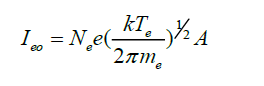(2)

Where Ieo is the random electron current and e, k, Vr, Te, and A are electron charge, Boltzmann constant, probe potential relative to Vs, electron temperature, and surface area of the probe, respectively. In reality, the current obtained in the electron retarding region includes both electron and ion currents.

### Langmuir Probe Working and Characteristics

The Langmuir probe technique involves applying a voltage to a metallic conductor immersed in plasma and observing the collected current. The observed total current, I, is a summation of various currents such as electron and ion thermal currents, photoelectron current, secondary electron currents, etc. The benefits of the simplicity of this technique, however, are offset by the complexity of the theory required to analyze the obtained current vs. potential or I-V, curves.

A typical current-voltage (I-V) curve for a Langmuir probe is shown in Figure. 3. It clearly shows the ion saturation region, the transition region, the electron saturation region, and the electron energy distribution region. These regions are used for evaluating the plasma parameters.

### Parts of the Langmuir I-V Curve

Ion saturation region

Biasing the probe increasingly with negative voltage, a point is reached where no electron is able to reach to the probe, only positive ions are collected. This region is called the ion saturation region and is used to determine the ion density and ion temperature of the plasma.

Electron transition region

Biasing the probe with negative voltage, the region where the probe starts to attract the electrons which are energetic enough to cross the potential barrier of the applied voltage is called the electron transition region. In this region, ions as well as some electrons are attracted towards the probe. The slope of the exponential transition region determines the electron temperature.

Electron saturation region

Biasing the probe with positive voltage, the electrons (and negative ions, if any) are attracted to the probe and electron saturation occurs. This region is called the electron saturation region and is used to determine the electron density of the plasma. The expression is used to calculate the electron density:(3)

where I is the saturation current, e is the electron charge, k is the Boltzmann’s constant, Te is the electron temperature, Mi is the mass of the ion, ne is the electron density, and A is the area of the probe.

Floating Potential

The potential, where no net current flows through the probe and there is a balance between electron and ion current (sufficient electrons to equate the ion and electron flux), giving zero net current is called the floating potential. It is the point on the current-voltage curve where the current drawn by the probe is zero.

Plasma Potential

When a straight line drawn from electron saturation region, where it intersects with the slope of the exponential part of the current-voltage I-V curve, the bias voltage corresponding to the intersection point gives the plasma potential. It is the potential acquired by isolated plasma.

### Electron Energy Distribution Function

The electron energy distribution function (EEDF) is proportional to the second derivative of the I-V curve. It gives a measure of the number density of the electrons as a function of the electron energy. It is useful to understand the process of formation of the plasma i.e., electron impact ionization and electron excitation.

### Theories of Spherical Langmuir Probe

Although many plasma diagnostic methods have been developed since the original work of Irving Langmuir, the Langmuir probe is still one of the most powerful plasma diagnostic tools due to its simplicity, low cost, adaptability, ability to determine the fundamental characteristics of plasma. A Langmuir probe may have any geometry, but cylindrical, spherical, and planar probes are usually employed because of their symmetry. The basis for Te measurements is the conventional Langmuir probe theory of Mott-Smith and Langmuir , assuming a Maxwellian energy distribution, F(E), given by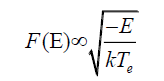(4)

where k is the Boltzmann constant. Thus, in a Maxwellian plasma, when the probe to plasma potential, V, is driven negative, Ie decreases exponentially(5)

where I0 is the random electron current given by ANe e(kTe/2πme) 1/2 , where e is the electron charge, me is electron mass, and A is the probe area. This equation for what is known as the electron retarding region in a Maxwellian plasma is the same for all probe geometries. This result has a generalization that the expression for the retarded current is identical for the three probe geometries for isotropic non-Maxwellian distributions. The derivation of Te is usually done by fitting the electron retarding region with an exponential function, and sometimes Te is obtained electronically by measuring the ratio of the first and second derivatives of Ie rather than through analysis of the I-V curves themselves.

The form of the acceleration region current depends on the geometry of the collector and is approximated by,(6)

where p = 0, 1/2, or 1 for planar, cylindrical, or spherical geometries, respectively. For Spherical Langmuir probe,(7)

For a Maxwellian plasma, all three probe geometries are suitable for the measurement of Te. However, the electron saturation regions differ greatly with collector geometry. The electron saturation region of the cylindrical probe is nearly independent of Te, whereas this region is highly temperature dependent for the planar and spherical probes. The cylindrical and spherical probes have the advantage of producing large saturation currents for the same density, an advantage for measurements in regions of very low density.

### Theoretical Considerations

According to Langmuir probe theory, Langmuir probe is a small conducting electrode and it can be a sphere, cylinder or planar. Generally the Langmuir probe inserts into plasma with a constant or time varying electric potential between the probe and the reference. As the charged plasma particles collide with the probe, then the probe draws electrical current which provides the condition of plasma. The amount of current flowing through the probe depends on the plasma parameters and the probe collecting area. The necessary conditions of Langmuir probe are:

1. The probe area should be small, in order to minimize the perturbation of the plasma.

2. The electrode dimension is larger in comparison to the Debye length (λD).

3. The electrons should obey the Maxwellian distribution.

The single spherical Langmuir probe sensor is schematically presented in figure below.

### The Orbital Motion Limit Theory

Langmuir and Mott-Smith have analyzed the current collection by cylindrical and spherical probes and named the limit of maximal current collection the Orbital-Motion-Limit. When the OML is valid, the ratio of the probe radius to Debye length is so small that the shielding becomes unimportant; at this limit, the number of electrons absorbed by the probe is determined by energy and angular momentum only. The Orbital-Motion-Limit theory has originally developed by assuming that the plasma is collision less, isotropic, there is no external magnetic field and the surface properties of the probe are homogeneous. Under OML conditions, the probe current is proportional to the plasma density and, at constant temperature; any density fluctuation can be measured by the relative variation of the probe current. None of the undisturbed plasma particles placed at infinity and capable of reaching the probe on the basis of its energy and angular momentum is excluded from doing so; as to say that there are no intervening barriers of active potential to block its motion.

The ion current flowing to the single spherical probe

From Figure. 4 the probe voltage, Vb applied to the spherical probe is negative with reference to the plasma potential, Vp. As a result, the spherical probe will attract positively charged ions to generate negative ion current, Ii. The ion current flowing from the plasma to the spherical probe with respect to the probe voltage is given by,

Figure 4: Schematic representation of a single spherical probe immersed in the plasma . A completely general theory describing the collection of charged particles by a probe does not exist. The appropriate theory depends on the parameters of the plasma and the size and shape of the probe. The modeling of the I-V characteristics of an ideal Langmuir single spherical probe is presented in the following sections.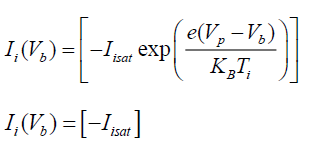(8)

When the ion temperature is equal to the electron temperature, i.e., Ti=Te, the ion-saturation current is(9)

Furthermore, when the ion temperature is much smaller than the electron temperature, i.e., Ti≪Te, the ion-saturation current is determined as the Bohm ion-saturation current.

The electron current flowing to the single spherical probe

When the probe voltage Vb applied to the spherical probe in Figure. 4 is positive with reference to the plasma potential, the spherical probe will attract negatively charged electrons to generate an electron current, Ie. The electron current flowing from the plasma to the spherical probe with respect to the probe voltage is: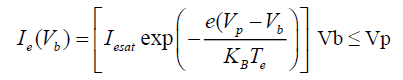(10)(11)

When the electron temperature is greater than the ion temperature, i.e., Te ≫ Ti the electron saturation current, Iesat is(12)

Electron Energy Distribution Function (EEDF)

Like any statistically characterized system, the free electrons in plasma are not all at the same energy. While the main force that initiates the electrons’, motion is the RF oscillation, a myriad of forces including collisions with other species in the plasma influences each electron and therefore the electrons take on a variety of speeds and corresponding energy levels. The electron energy distribution function (EEDF) plays an important role in plasma modeling . It has important consequences in calculating the rate coefficients of electron induced processes, such as ionization and excitation. Various approaches can be used to describe the EEDF, such as Maxwellian, Druyvesteyn, or using a solution of the Boltzmann equation. The electron energy distribution function in low pressure discharges may often be approximated by either Maxwellian or Druyvesteyn distribution.

The Relationship between Electron Current and EEDF

As an electron approaches a surface with a retarding potential Vp, it will either be collected or repelled based on its velocity vector relative to the retarding potential. If the perpendicular component of the velocity provides sufficient energy to overcome the potential, the electron is collected. By assuming isotropic distributions, one can integrate over all possible incident angles, accounting for the “perpendicular energy component” relative to the potential, and obtain an equation for electron current.

In 1930, the more commonly used “Druyvesteyn Relation” was derived for spherical probes.(13)

Or,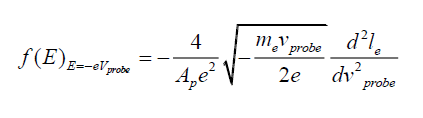(14)

Where f(E) is the electron energy distribution function, Ap is the surface area of the probe (typically approximation for a spherical probe by 4πr2 p ), e is the electron charge, me is the electron mass, Vprobe is the probe voltage and Ie is the electron current through the probe circuit. This was only derived for the spherical geometry. The cylindrical geometry does not have a nice analytical differential from like the spherical solution does.

### Maxwellian Electron Energy Distribution Function

For any group of particles distributed throughout space, the distribution of the particles can be described by the local number density at each point within the defined space . If a gas is in thermodynamic equilibrium, then the particle distribution tends to the Maxwellian distribution of particles.

It is now possible to write the normalized energy distribution function,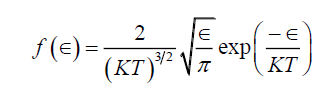(15)

Maxwellian EEDF can be obtained from these equations,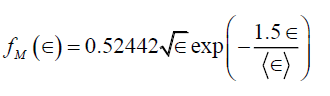(16)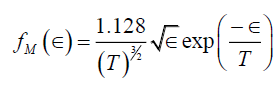(17)

### Results and Discussion

The Langmuir probe technique for in situ measurement of plasma parameters has been around for eight decades, deriving the parameters with accuracy from the data acquired by a Langmuir probe immersed in space plasma is still a challenging task. In the present thesis work theoretical study of Spherical Langmuir Probe in Maxwellian plasma are studied. This work is typically based on the I-V characteristic of Spherical Langmuir Probe . The main propose of this work is to study the Current-Voltage characteristic of spherical Langmuir probe with different temperatures and densities. The methodology of our study includes the theoretical derivation of the relationships between various parameters and then plotting the parameters in suitable range from a mathematical software (here MATLAB is employed). While plotting the graphs, we have use some standard values of constants such as mass and charge of electrons, Boltzmann constant etc.

The variation of spherical Langmuir Probe I-V curve with temperature

The I-V characteristic is a fundamental part of diagnosis of plasma parameters. So, it is depicted in the following figure. For this purpose, it is assumed that for density, Ne =1016 m-3 , radius of spherical probe (rp=2.5 mm). These figures illustrate the effect of Te on the width of the electron retardation regions. Here, Figure. 5 depicts that the spherical probe curve computed for a Maxwellian plasma illustrate the effect of Te = 0.13 eV on the width of the retarding region. The ion saturation region is flat because the calculation assumes an infinite plane; i.e., a perfect guarded spherical probe [13-15].

Figure 5: The spherical Langmuir Probe I-V curve computed for a Maxwellian plasma at Te= 0.13 eV.

The amplitude of the ion saturation current varies greatly with Te.. When the plasma temperature is 0.13 eV the knee of the curve is sharp and is a good measure of Vs. The Floating Potential Vf is nearly -0.7 volt. This floating potential is typically negative because mobile electrons tend to strike the probe more frequently than positive ions. The knee occurs when the probe has been saturated with electrons, causing additional electrons to be repelled [16,17]. Plasma potential Vs are greater than 0.2 volt. For probe potential is greater than plasma potential (Vp > Vs), Ie increases slowly as the collection area grows due to an increase in sheath thickness. In the Transition Region, the ion current is negligible, and the electrons are partially repelled by the negative potential Vp−Vs. In a Maxwellian plasma, this part of the curve is exponential as shown in above figure.

When the plasma temperature is 0.26 eV the knee of the curve is little bit sharp. In Figure. 6 the Floating Potential Vf is nearly -1.5 volt this shows that when we gradually increase the probe potential greater than floating potential we get the current exponentially increasing in the Maxwellian plasma.

Figure 6: The spherical Langmuir probe I-V curve computed for a Maxwellian plasma at Te= 0.26 eV.

The space potential Vs is near the upper knee of the curve. At the far left, where all the electrons have been repelled, we have the Ion Saturation current, Isat. When Vp >> Vs, an electron current Ie is collected and when Vp << Vs, an ion current Ii is collected.

In Figure. 7 the knee is just a small bend occurring when the electron temperature is 0.52 eV as comparative to the lower temperatures. The Floating Potential Vf is nearly -3 volt where the ion and electron currents are equal, and the net current is zero. At 0.52 eV plasma temperatures, the floating potential is less as comparative to other lower temperature. This means while increasing the probe voltage greater than Vf = -3 volt then probe current gradually increasing [18-20]. The exponential growth of Ie with Vp should continue until Vp=Vs, when none of the electrons is repelled by a negative potential. The electron saturation region of the Spherical Probe is highly dependent of Te, so that it cannot apply on the fixed probe potential to make continuous measurements of Ne i.e., probe potential should be varied.

Figure 7: The spherical Langmuir probe I-V curve computed for a Maxwellian plasma at Te= 0.52 eV.

The variation of spherical Langmuir Probe I-V curve for various densities

The I-V characteristic curves of Spherical Langmuir Probe in Maxwellian plasmas for various densities are given below. Which shows the variation of I-V characteristic with different number density of electron Ne with constant temperature [21,22]. The calculation assumed radius of spherical probe (rp=2.5 mm), temperature Te= 0.52 eV and the number density of electron Ne are 1016 m-3, 1015 m-3, 1014 m-3 respectively.

Here Figure. 8 shows that as the electron temperature increases width of the curve also increases and vice-versa. For the smaller the value of temperature energy distribution raising sharply after the certain value of energy it decreases slightly [23-25]. This curve shows that electron temperature of the plasma does affect the electron energy distribution function. When the electron temperature of the plasma is Te= 0.26 eV distribution is concentrated at low energies, whereas at Te= 0.52 eV the distribution shows electrons are scattered much higher energies too with a tail extending to energy of electron 3.5 eV. The simulation of the Maxwellian distribution shown in Figure. 4 and Figure. 5 matches up very well in shape but loser the density profile for temperatures out to Te= 0.52 eV. For energies, greater than Te, the overall shape of the distribution is maintained, but with a slightly elevated tail temperature is obtained [26-28].

Figure 8: Comparison of Maxwellian electron energy distributions in different ranges of temperature Te=3000 K (0.26 eV) and 6000 K (0.52 eV).

### Conclusion

The I-V characteristic of the Spherical Langmuir Probe in the Maxwellian Space plasma at different temperature range are plotted in graph by using the mathematical software by putting the suitable ranges for various parameters. All elementary processes were extensively discussed and most of information has been presented. With the help of the (volt–ampere curves) of spherical Langmuir probes, the different parameters of plasma can be determined such as plasma potential, floating potential, probe currents in different probe voltage and so on. And the effect of temperature Te on the width of the electron retardation regions is clearly studied in this dissertation. Thus, measured plasma parameters, and the relationship between the densities of the plasma on probe current, effect of temperature on I-V curve, are of fundamental importance for research on laboratory plasma, ionosphere terrestrial plasma and industrial application. The electron temperature of the plasma effect the Maxwellian electron energy distribution function (EEDF). Maxwellian EEDF function curve demonstrates that at low energy (less than Te), the calculated distribution presents a significantly underestimated density profile. At higher energy range, the shape of the distribution recovers and the tail trend with energy is maintained and decreases exponentially. The knowledge of the real EEDF is of great importance in understanding the underlying physics of processes occurring at the plasma edge in tokamaks, such as the formation of transport barriers, plasma-wall interactions, and edge plasma turbulence.

### References

Welcome Message

###### Citations : 252

Journal of Physics & Astronomy received 252 citations as per Google Scholar report

#### Indexed In

• Open J Gate
• China National Knowledge Infrastructure (CNKI)
• Cosmos IF
• Directory of Research Journal Indexing (DRJI)
• MIAR
• Secret Search Engine Labs
• Euro Pub